# Class 12 Maths NCERT Solutions for Chapter 7 Integrals Exercise 7.6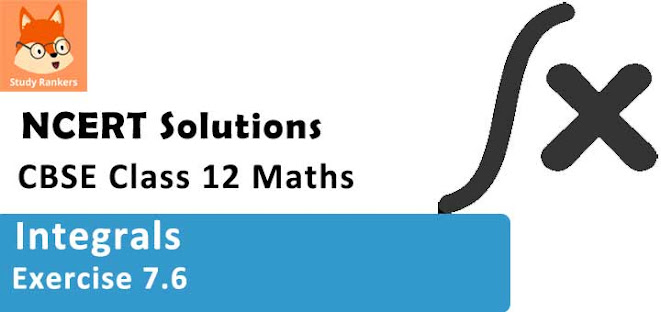### Integrals Exercise 7.6 Solutions

1. Integrate the functions x sin x

Solution

Let l = ∫x sin x dx
Taking x as first function and sin x as second function and integrating by parts, we obtain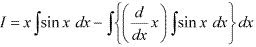= x(-cos x) - ∫1.(- cos x) dx
= -x cos x + sin x + C

2. Integrate the functions x sin 3x

Solution

Let I = ∫x sin 3x dx
Taking x as first function and sin 3x as second function and integrating by parts, we obtain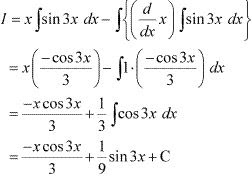3. Integrate the functions x2 ex.

Solution

Let I = ∫ x2 ex dx
Taking x2 as first function and ex as second function and integrating by parts, we obtain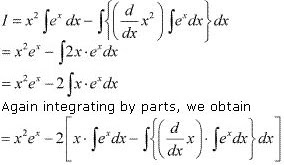= x2 ex - 2[xex - ∫ex dx]
= x2 ex - 2[xex - ex ]
= x2 ex - 2xex + 2ex + C
= ex (x2 - 2x + 2) + C

4. Integrate the functions x log x

Solution

Let I = ∫ x log x dx
Taking log x as first function and x as second function and integrating by parts, we obtain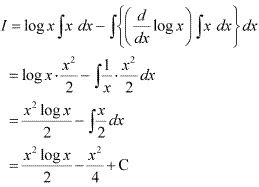5. Integrate the functions x log 2x

Solution

Taking log 2x as first function and x as second function and integrating by parts, we obtain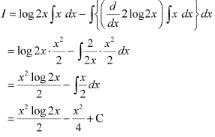6. Integrate the functions x2 log x

Solution

Let I = ∫x2 log x dx
Taking log x as first function and x2 as second function and integrating by parts, we obtain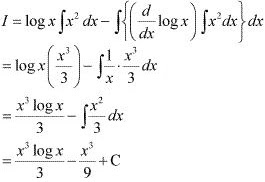7. Integrate the functions x sin-1 x

Solution

Taking sin-1 x as first function and x as second function and integrating by parts, we obtain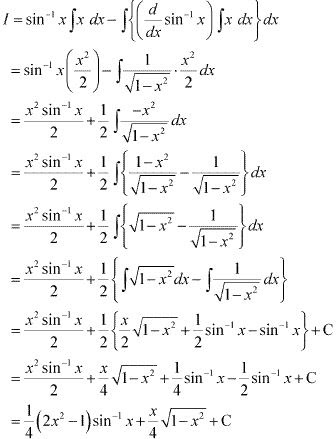9. Integrate the functions x cos-1 x

Solution

Let I =  ∫x cos-1 x dx
Taking cos-1 x as first function and x as second function and integrating by parts, we obtain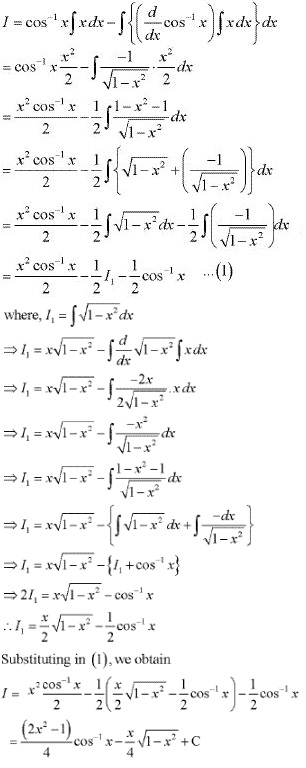10. Integrate the functions (sin-1 x)2
Solution
Let I = ∫(sin-1 x)2 .l dx
Taking (sin-1 x)2 as first function and 1 as second function and integrating by parts, we obtain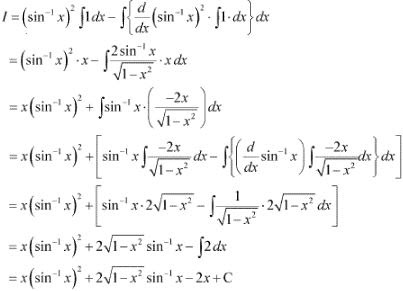11. Integrate the functions (x cos-1 x)/√(1 - x2 ) .
Solution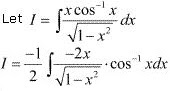Taking cos-1 x as first function and [-2x/√(1 - x2)] as second function and integrating by parts, we obtain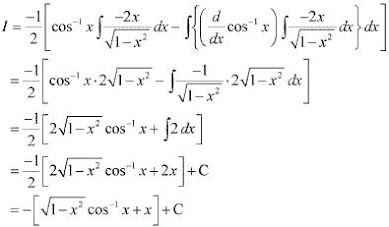12. Integrate the functions x sec2 x.
Solution
Let I = ∫x sec2 x dx
Taking x as first function and sec2 x as second function and integrating by parts, we obtain= x tan x -  ∫1 . tan x dx
= x tan x + log |cos x| + C

13. Integrate the functions tan-1 x.
Solution
Let I = ∫1. tan-1 x dx
Taking tan-1 x as first function and 1 as second function and integrating by parts, we obtain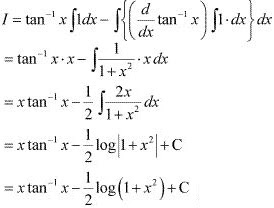14. Integrate the functions x (log x)2
Solution
I = ∫ x (log x)2 dx
Taking (log x)2 as first function and x as second function and integrating by parts, we obtain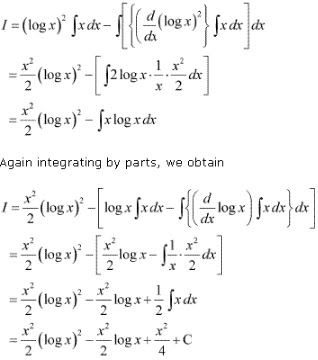15. Integrate the functions (x2 + 1) log x
Solution
Let I = ∫(x2 + 1) log x dx = ∫x2 log x dx + ∫log x dx
Let I = I1 + I2 …(1)
Where, I1 = ∫x2 log x dx and I2 = ∫ log x dx
I1 = ∫x2 log x dx
Taking log x as first function and x2 as second function and integrating by parts, we obtain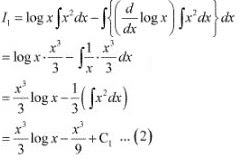I2 = ∫ log x dx
Taking log x as first function and 1 as second function and integrating by parts, we obtain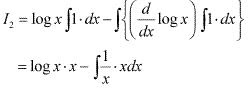= x log x - ∫1 dx
= x log x - x + C2 ...(3)
Using equation (2) and (3) in (1), we obtain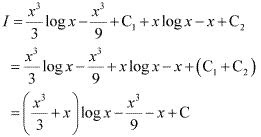16. Integrate the functions ex (sinx + cosx)
Solution
Let I =  ∫ex (sin x + cos x) dx
Let f(x) = sin x
⇒ f'(x) = cos x
⇒ I = ∫ ex {f(x) + f '(x)} dx
It is known that,  ∫ ex {f(x) + f '(x)} dx = ex f(x) + C
∴ I = ex sin x + C

17. Integrate the functions xex /(1 + x)2
Solution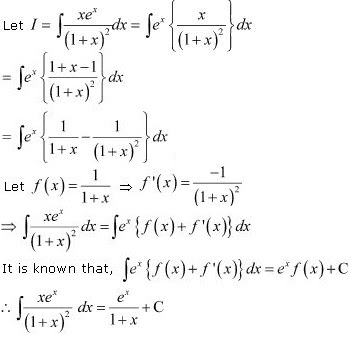18. Integrate the function ex [(1 + sinx)/(1 + cos x)]
Solution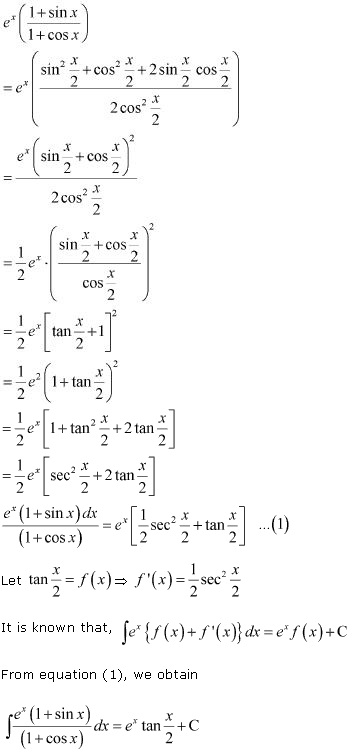19. Integrate the functions ex (1/x - 1/x2 )
Solution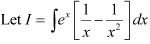Also, let 1/x = f(x) ⇒ f '(x) = -1/x2
It is known that, ∫ ex {f(x) + f '(x)}dx = ex f(x) + C
∴ I = ex /x + C

20. Integrate the functions (x - 3)ex /(x - 1)3
Solution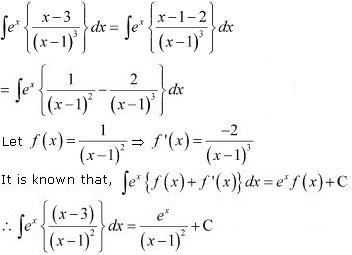21. Integrate the functions e2x sin x
Solution
Let I = ∫ e2x sin x dx  ...(1)
Integrating by parts, we obtain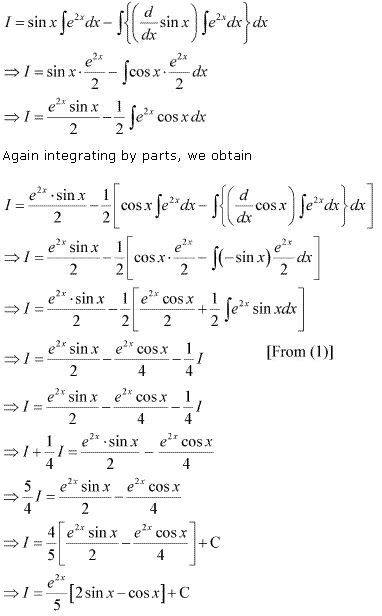22. Integrate the functions sin-1 [2x/(1 + x2)]
Solution
Let x = tan θ
⇒ dx = sec2 θ dθ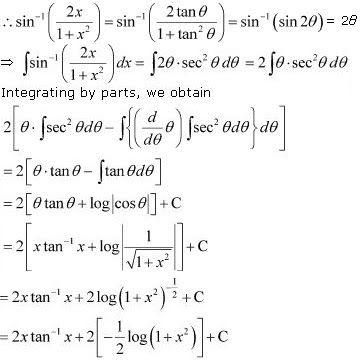23. Choose the correct answer ∫ x2 ex3 dx equals
(A) (1/3) ex3 + C
(B) (1/3) ex2 + C
(C) (1/2) ex3 + C
(D) (1/3) ex2 + C
Solution
Let I = ∫ x2 ex3 dx
Also, let x3 = t ⇒ 3x2 dx = dt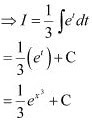Hence, the correct answer is A.

24. Choose the correct answer ∫ ex sec x(1 + tan x) dx equals
(A) ex cos x + C
(B) ex sec x + C
(C) ex sin x + C
(D) ex tan x + C
Solution
∫ ex sec x(1 + tan x) dx
Let I = ∫ ex sec x(1 + tan x) dx = ∫ ex (sec x + sec x tan x) dx
Also, let sec x = f(x) ⇒ sec x tan x = f '(x)
It is known that, ∫ ex {f(x) + f '(x)} dx = ex f(x) + C
∴ I = ex sec x + C
Hence, the correct answer is B.